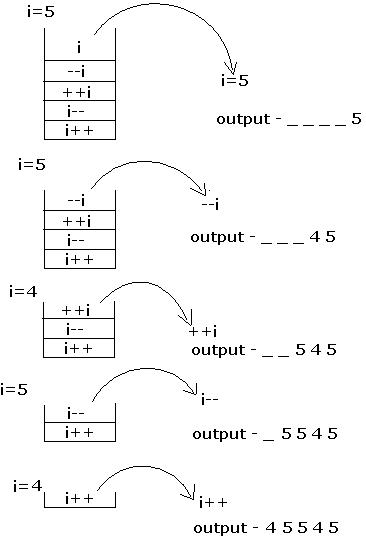# Determine Output: ``````void main() { int i=5; printf("%d%d%d%d%d", i++, i--, ++i, --i, i); }``````

A. 45545

B. 54544

C. 55445

D. 54554

### Solution(By Examveda Team)

The arguments in a function call are pushed into the stack from left to right. The evaluation is by popping out from the stack. and the evaluation is from right to left, hence the result. See the picture below1.we are compile this question but answer is change and not given in options

2.You are not using stack ,i am getting 45555 answer please respond

3.Ok the stack fact

4.exactly how a could be the answer

5.how option A is the answer?

Related Questions on C Miscellaneous